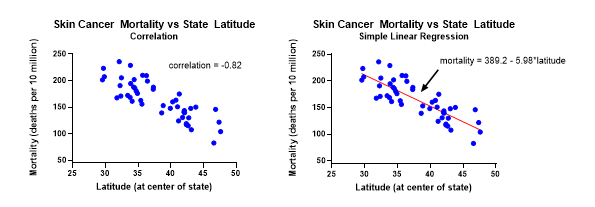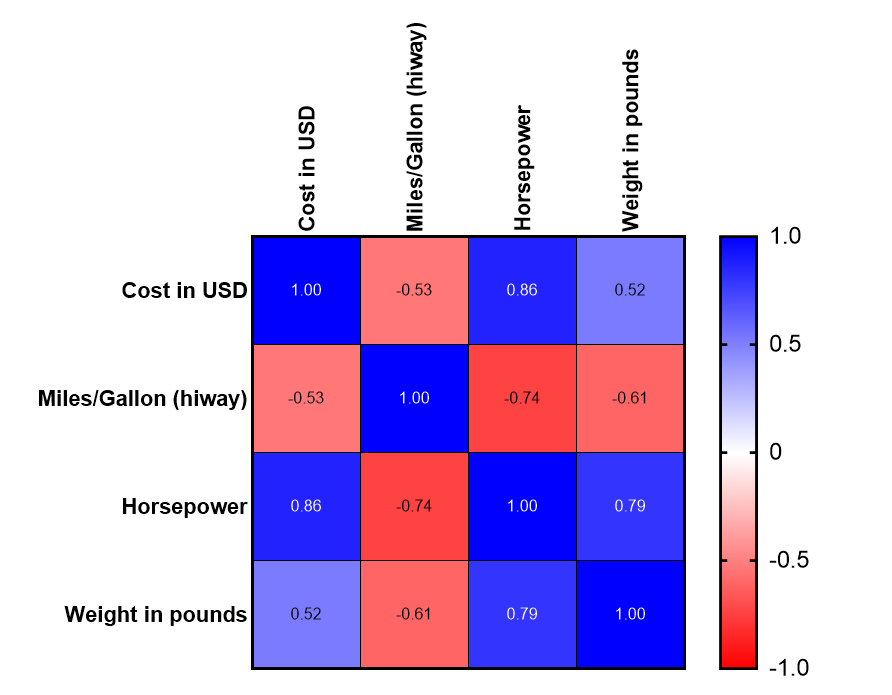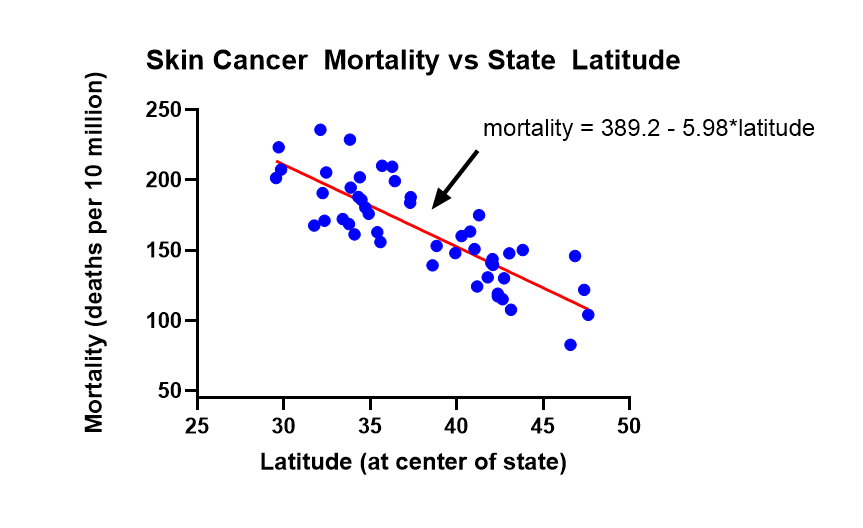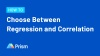KNOWLEDGEBASE - ARTICLE #1141

# What is the difference between correlation and linear regression?

When investigating the relationship between two or more numeric variables, it is important to know the difference between correlation and regression. The similarities/differences and advantages/disadvantages of these tools are discussed here along with examples of each.

Correlation quantifies the direction and strength of the relationship between two numeric variables, X and Y, and always lies between -1.0 and 1.0. Simple linear regression relates X to Y through an equation of the form Y = a + bX.Key similarities

• Both quantify the direction and strength of the relationship between two numeric variables.
• When the correlation (r) is negative, the regression slope (b) will be negative.
• When the correlation is positive, the regression slope will be positive.
• The correlation squared (r2 or R2) has special meaning in simple linear regression. It represents the proportion of variation in Y explained by X.

Key differences

• Regression attempts to establish how X causes Y to change and the results of the analysis will change if X and Y are swapped. With correlation, the X and Y variables are interchangeable.
• Regression assumes X is fixed with no error, such as a dose amount or temperature setting. With correlation, X and Y are typically both random variables*, such as height and weight or blood pressure and heart rate.
• Correlation is a single statistic, whereas regression produces an entire equation.

*The X variable can be fixed with correlation, but confidence intervals and statistical tests are no longer appropriate. Typically, regression is used when X is fixed.

• Correlation is a more concise (single value) summary of the relationship between two variables than regression. In result, many pairwise correlations can be viewed together at the same time in one table.

• Regression provides a more detailed analysis which includes an equation which can be used for prediction and/or optimization.

# Correlation Example

As an example, let’s go through the Prism tutorial on correlation matrix which contains an automotive dataset with Cost in USD, MPG, Horsepower, and Weight in Pounds as the variables. Instead of just looking at the correlation between one X and one Y, we can generate all pairwise correlations using Prism’s correlation matrix. If you don’t have access to Prism, download the free 30 day trial here. These are the steps in Prism:

1. Open Prism and select Multiple Variables from the left side panel.
3. Click Create.
4. Click Analyze.
5. Select Multiple variable analyses > Correlation matrix.
6. Click OK twice.
7. On the left side panel, double click on the graph titled Pearson r: Correlation of Data 1.The Prism correlation matrix displays all the pairwise correlations for this set of variables.

• The red boxes represent variables that have a negative relationship.
• The blue boxes represent variables that have a positive relationship
• The darker the box, the closer the correlation is to negative or positive 1.
• Ignore the dark blue diagonal boxes since they will always have a correlation of 1.00.

Key findings:

• Horsepower and MPG have a strong negative relationship (r = -0.74), higher horsepower cars have lower MPG.
• Horsepower and cost have a strong positive relationship (r = 0.88), higher horsepower cars cost more.

Note that the matrix is symmetric. For example, the correlation between “weight in pounds” and “cost in USD” in the lower left corner (0.52) is the same as the correlation between “cost in USD” and “weight in pounds” in the upper right corner (0.52). This reinforces the fact that X and Y are interchangeable with regard to correlation. The correlations along the diagonal will always be 1.00 and a variable is always perfectly correlated with itself.

When interpreting correlations, you should be aware of the four possible explanations for a strong correlation:

• Changes in the X variable causes a change the value of the Y variable.
• Changes in the Y variable causes a change the value of the X variable.
• Changes in another variable influence both X and Y.
• X and Y don’t really correlate at all, and you just happened to observe such a strong correlation by chance. The P value quantifies the likelihood that this could occur.

# Regression Example

The strength of UV rays varies by latitude. The higher the latitude, the less exposure to the sun, which corresponds to a lower skin cancer risk. So where you live can have an impact on your skin cancer risk. Two variables, cancer mortality rate and latitude, were entered into Prism’s XY table. The Prism graph (right) shows the relationship between skin cancer mortality rate (Y) and latitude at the center of a state (X). It makes sense to compute the correlation between these variables, but taking it a step further, let’s perform a regression analysis and get a predictive equation.The relationship between X and Y is summarized by the fitted regression line on the graph with equation: mortality rate = 389.2 - 5.98*latitude.  Based on the slope of -5.98, each 1 degree increase in latitude decreases deaths due to skin cancer by approximately 6 per 10 million people.

Since regression analysis produces an equation, unlike correlation, it can be used for prediction. For example, a city at latitude 40 would be expected to have 389.2 - 5.98*40 = 150 deaths per 10 million due to skin cancer each year.Regression also allows for the interpretation of the model coefficients:

• Slope: every one degree increase in latitude decreases mortality by 5.98 deaths per 10 million.
• Intercept: at 0 degrees latitude (Equator), the model predicts 389.2 deaths per 10 million. Although, since there are no data at the intercept, this prediction relies heavily on the relationship maintaining its linear form to 0.

In summary, correlation and regression have many similarities and some important differences. Regression is primarily used to build models/equations to predict a key response, Y, from a set of predictor (X) variables. Correlation is primarily used to quickly and concisely summarize the direction and strength of the relationships between a set of 2 or more numeric variables.

The table below summarizes the key similarities and differences between correlation and regression.

 Topic Correlation Regression When to use For a quick and simple summary of the direction and strength of pairwise relationships between two or more numeric variables. To predict, optimize, or explain a numeric response Y from X, a numeric variable thought to influence Y. Quantifies direction of relationship Yes Yes Quantifies strength of relationship Yes Yes X and Y interchangeable Yes No Y Random Yes Yes X Random Yes No Prediction and Optimization No Yes Equation No Yes Extension to curvilinear fits No Yes Cause and effect No Attempts to establish# Test your understanding of Correlation and Regression

Which tool, correlation or regression, would you use in each of these scenarios:

1. You have two measuring systems and you want to see how well they agree with each other. So you measure the same 20 parts with each measuring system.
2. You want to predict blood pressure for different doses of a drug.
3. A clinical trial has multiple endpoints and you want to know which pair of endpoints has the strongest linear relationship.
4. You want to know how much the response (Y) changes for every one unit increase in (X).